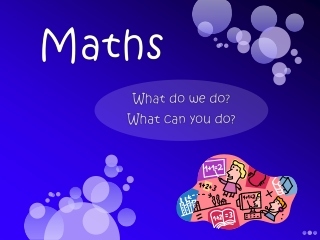DownloadDownload PresentationSIMPLIFICATION

# SIMPLIFICATION

Download Presentation## SIMPLIFICATION

- - - - - - - - - - - - - - - - - - - - - - - - - - - E N D - - - - - - - - - - - - - - - - - - - - - - - - - - -
##### Presentation Transcript

1. Maths What do we do? What can you do?

2. Some people love it; some people hate it. But everyone has to do it. It came naturally to me Painful Struggle Success MATHS Battle Boring Pages of sums Hard work Easy When I was taught by Mr Hardy I could do it Enjoyable

3. How do our children learn in Maths lessons at Orleans? • Lots of talking • Thinking • Self-discovery • Problem solving • Asking questions • Real-life learning • Practical and engaging lessons – fascinators!CB's • ‘I hear and I forget. I see and I remember. I do and I understand.’ • (A Chinese proverb)

4. ‘It just doesn’t look like it did in my day.’

5. This is the way we do it! Why use a number line? Mental Calculations Written Calculations

6. Mental Starter How many numbers can you make using these digits? What’s the biggest number you can make?

7. Place Value • One of the most important concepts for children to understand H T U 6 60 600 Activity 2

8. Hundred Square -1 +1 Addition Subtraction Multiplication Division + 10 12 + 23 = 35

9. Early stages of + and -

10. Same problem, different methods…

11. Partitioning + 87 34 + 53 = 30 50 3 4 30 + 50 = 80 4 + 3 = 7 80 + 7 = 87

12. Number line + + 10 +1 +1 36 37 26 38 38 26 + 12 = + 10 + 20 +5 +1 42 62 72 77 78 42 + 36 = 78

13. Number line - • Subtraction as taking away - 10 - 5 - 2 30 15 20 13 30 – 17 = 13

14. Number line - • Subtraction as finding the difference • (starting from the lower number and counting on to the largest) 20 – 7 = ? +3 + 10 7 10 20 Jump to next multiple of 10 Count the jumps 10 + 3 = 13 20 – 7 = 13

15. Column Addition- Stage 1 35 54 80 9 89 • Add 10s first the units • Building on mental calculation strategies: adding biggest number first +

16. Multiplication End of year expectations… Year 1 Count in steps of 2, 5 and 10 Year 2 Recall multiplication facts for the 2, 5 and 10 x tables Year 3 Recall multiplication facts for the 3, 4 and 6 x tables

17. What’s the best way to learn your tables?

18. Percy Parker Up and down the stairs YouTube Clapping, jumping, passing a ball etc Chanting or Singing www.learnyourtimestables.com

19. Magnetic x tables Building up the x table number sentences ‘Know Your’ – www.ko-box.com Reading and writing them Flash cards Missing number sentences Speed Tests

20. Hundred Square Find patterns in the numbers e.g. all even/all end in.../first digit goes up, last digit goes down X9 If you add the answer’s digits together, they add 9 e.g. 9 x 5 = 45 (4 + 5 = 9) Find Patterns If you know your 2x tables, then you can work out 4x any number. X by 2, then double it. 7 x 8 (always a tricky one!) Answer first: 56 = 7 x 8. So it’s 5, 6, 7, 8. Easy!

21. Online games – endless websites. See handouts for some. Multiplication War – pack of playing cards X table apps on your iphone Games Board Games Origami Fortune Teller Snap

22. What’s the best way to learn your tables? • There isn’t a truly pain-free answer because… • Everyone learns in best in different ways • Real fluency demands practise over • weeks and months • Drilling is the key! • Practise, practise, practise!

23. Early stages of multiplication

24. Number line x Multiplying as repeated addition 2 x 4 +2 +2 +2 +2 0 2 4 6 8 2 x 4 = 2 + 2 + 2 + 2 So, 2 x 4 = 8

25. Arrays x 3 x 6 Or Add the dots

26. What multiplication are these arrays showing?

27. Partitioning x 25 x 3 = 75 20 5 x 3 60 15 = 75

28. Division Sharing Grouping

29. Number line 4 groups of 3 + 3 + 3 + 3 + 3 9 12 6 0 3 Add the jumps = 4 12 3 = 4

30. Whilst children learn about numbers and maths at school, there are also lots of ways that you can support your child at home. It doesn’t have to be by doing pages of sums or text books – there are lots of fun activities and games you can do or include in your everyday routines! Here are a few ideas to help you…

31. ‘How to do Maths so your children can too’ by Naomi Suni Learning Targets for Year 1 Learning Targets for Year 2